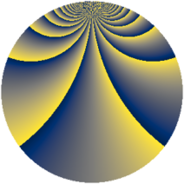# Properties

 Label 1600.2.bxLevel $1600$ Weight $2$ Character orbit 1600.bx Rep. character $\chi_{1600}(223,\cdot)$ Character field $\Q(\zeta_{20})$ Dimension $480$ Sturm bound $480$

# Related objects

## Defining parameters

 Level: $$N$$ $$=$$ $$1600 = 2^{6} \cdot 5^{2}$$ Weight: $$k$$ $$=$$ $$2$$ Character orbit: $$[\chi]$$ $$=$$ 1600.bx (of order $$20$$ and degree $$8$$) Character conductor: $$\operatorname{cond}(\chi)$$ $$=$$ $$200$$ Character field: $$\Q(\zeta_{20})$$ Sturm bound: $$480$$

## Dimensions

The following table gives the dimensions of various subspaces of $$M_{2}(1600, [\chi])$$.

Total New Old
Modular forms 2016 480 1536
Cusp forms 1824 480 1344
Eisenstein series 192 0 192

## Trace form

 $$480q + O(q^{10})$$ $$480q - 24q^{17} + 24q^{25} + 24q^{65} + 24q^{73} + 120q^{81} + 120q^{89} - 360q^{97} + O(q^{100})$$

## Decomposition of $$S_{2}^{\mathrm{new}}(1600, [\chi])$$ into newform subspaces

The newforms in this space have not yet been added to the LMFDB.

## Decomposition of $$S_{2}^{\mathrm{old}}(1600, [\chi])$$ into lower level spaces

$$S_{2}^{\mathrm{old}}(1600, [\chi]) \cong$$ $$S_{2}^{\mathrm{new}}(200, [\chi])$$$$^{\oplus 4}$$$$\oplus$$$$S_{2}^{\mathrm{new}}(800, [\chi])$$$$^{\oplus 2}$$